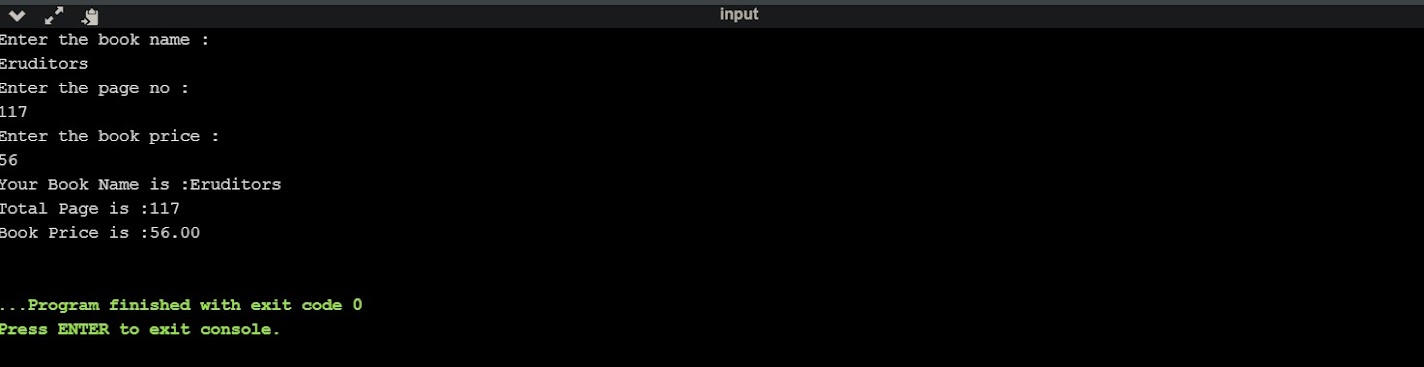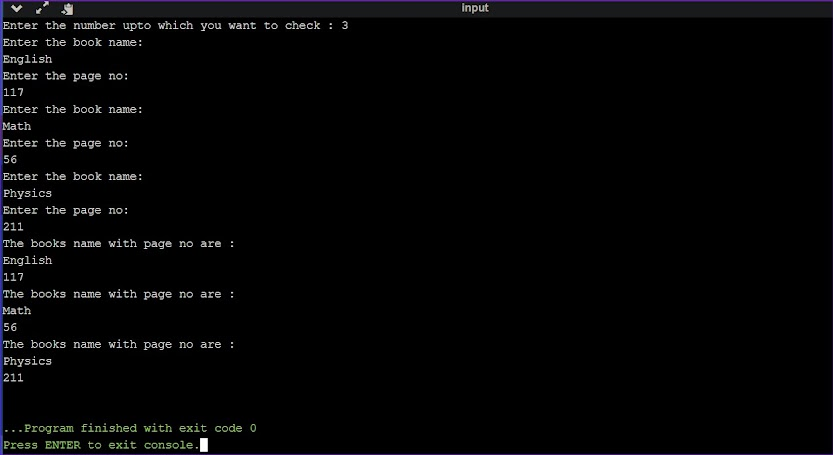What is Structure in C

Structure is basically a user-defined data type that can store logically related information of different data types.

Why do we need Structure ?

1. It is used to create a clubbed data type which contains different data types, like int, char, float etc.
2. It helps us to organize a complex data in a meaningful way.

Difference between Array and Structure :

Main difference is an Array can store only the information of same data type, in other hand a structure can store information of different data types.

How to create a Structure ?

Syntax :
struct structureName
{
datatype member1;
datatype member2;
...
};
Example :
struct Student
{
char Name;
int Roll;
float Marks;
};
//"struct" as keyword for structure
//"student" as structure tag and name

What is member ?

Individual structure elements are called as “members”.

How to define Structure Variables ?

We can define structure variables in two way :
1st way :
struct Student
{
char Name;
int Roll;
float Marks;
};
struct Student s1,s2;
2nd way :
struct Student
{
char Name;
int Roll;
float Marks;
}s1,s2;

How to access members of Structure ?

We can access structure members in two ways :

1. Member or Dot Operator :

This operator used to access members of structure.
Eg. s1.Name
Example :
//Write a program in C++ to declare and print structure named student with variable student_name, roll, marks, grade.

#include<iostream>
using namespace std;
int main()
{
struct Student
{
char student_name;
int roll;
float marks;
};
/* struct student s1;
s1.roll = 117; s1.marks = 56.47; */
struct Student s1 = {"Arijit Roy",117,56.47,"B"};
cout <<"Given details are : ";
cout <<"Name : "<<s1.student_name <<endl <<"Roll : " <<s1.roll <<endl <<"Marks : " <<s1.marks <<endl <<"Grade : " <<s1.grade <<endl;
return 0;
}

Output :2. Structure Pointer Operator :

This operator used to access pointer in a structure.
Eg. p-->name
Example :
//Write a program in C++ to declare and print structure named Book with variables bookname, page number and price (using pointer)

#include<iostream>
using namespace std;
int main ()
{
struct book
{
char name;
int pageno;
float price;
};
struct book b;
cout << "Enter the book name :" << endl;
cin >> b.name;

cout << "Enter the page no :" << endl;
cin >> b.pageno;

cout << "Enter the book price :" << endl;
cin >> b.price;
struct book *p;
p = &b;
cout << "Your Book Name is :" << p->name << endl << "Total Page is :" << p->pageno << endl << "Book Price is :" << p->price << endl;
return 0;
}

Output :What is an Array of structures ?

By name, Array of structures means it is a contiguous memory location, that will store value of structure data type. So that array of structure also known as the collection of structure.

Sample Program :
//Write a program in C++ to declare a structure array of Book with variable book name, page number.

#include<iostream>
using namespace std;
int main()
{
struct book
{
char name;
int page_no;
};
int i,size;
cout <<"Enter the number upto which you want to check : ";
cin >>size;
struct book b[size];		//using array
for (i = 0; i < size; i++)
{
cout <<"Enter the book name: " <<endl;
cin >> b[i].name;
cout <<"Enter the page no: " <<endl;
cin >> b[i].page_no;
}
for (i = 0; i < size; i++)
cout << "The books name with page no are : " <<endl <<b[i].name <<endl <<b[i].page_no <<endl;
return 0;
}
Output :Limitations of C++ Structure :

1. Two structure variables can not be compared for equality or inequality.
2. C++ structures do not permit functions inside structure.